M340L Second midterm exam solutions, March 7, 2003

1. Consider the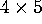matrix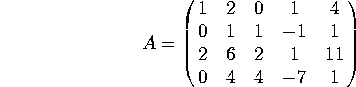a) What is the rank of A? [Note: the row reduction of A can be done using integers only. If you start getting fractions, you probably made a mistake]

After row reduction, A becomes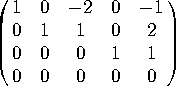. There are 3 pivots, so the rank is three.

b) Find a basis for the column space of A.

The pivots are in the 1st, 2nd and 4th columns, so the 1st, 2nd and 4th columns of the ORIGINAL matrix form a basis for Col(A):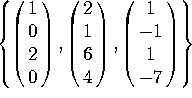c) Find a basis for the null space of A.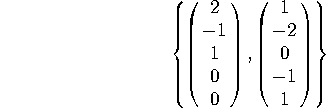2. Consider the linear transformation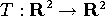defined by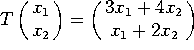.

a) Find the matrix of this linear transformation.

The matrix is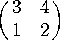, whose determinant is 2.

b) Is T one-to-one? Is T onto?

Since the determinant is nonzero, the matrix is invertible, and T is both 1-1 and onto.

c) Let S be a rectangle whose area is 2. What is the area of T(S)?

The determinant is the expansion factor for area (or, in higher dimensions, volume), so the area of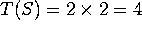.

3. Compute the following determinants:

a)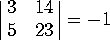b)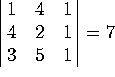c)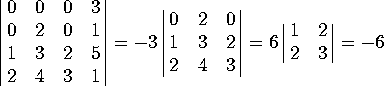4. Consider the matrix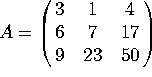, that factorizes as A = LU, where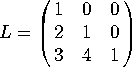and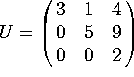. Let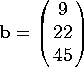. Solve the problem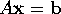in two steps (Note: you do NOT get any credit for solving the full equations directly):

a) Solve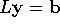for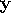, and then

By row reducing the augmented matrix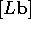we get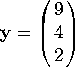.

b) Solve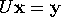for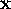. Does this solution also satisfy?

By row reducing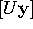we get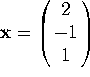. Multiplying out Ax does indeed give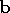. You can see this either by explicit multiplication, or since A x = LUx = L(Ux) = Ly = b.

5. Indicate whether each of these statements is true or false. If a statement is sometimes true and sometimes false, write ``false''. You do NOT have to justify your answers. There is no penalty for wrong answers, so go ahead and guess if you are unsure of your answer.

a) The rank of a matrix is the dimension of its null space.

False. The rank is the dimension of the COLUMN space.

b) Swapping two rows of a matrix does not change the determinant of that matrix.

False. Swapping two rows changes the sign of the determinant.

c) If A and B are square matrices of the same size, then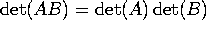.

True.

d) If an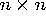matrix has an inverse, then the columns of that matrix span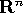.

True.

e) If the determinant of a (square) matrix is zero, then its columns are linearly dependent.

True. Conversely, if the determinant is nonzero, then the columns are linearly independent.

f) The span of 3 vectors inis a 3-dimensional subspace of.

False. The three vectors may be linearly dependent, in which case their span would have dimension less than 3.

g) Let A be anmatrix. The dimension of Col(A) plus the dimension of Null(A) equals 5.

False. The dimension of Col(A) plus the dimension of Null(A) equals 7.

h) If two vectors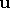and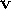lie in a subspace H of, then every linear combination ofandalso lies in H.

True.

i) If a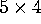matrix has rank 3, then its null space is 1-dimensional.

True.

j) A basis for a subspace H is a linearly independent set of vectors whose span is H.

True.